# TSA Resampling VI

LabVIEW 2014 Advanced Signal Processing Toolkit Help

Edition Date: June 2014

Part Number: 372656C-01

»View Product InfoDownload Help (Windows Only)

Owning Palette: Preprocessing VIs

Requires: Advanced Signal Processing Toolkit

Resamples the equally- or unequally-sampled time series into a new time series with a specified time interval using the interpolation method. Wire data to the Xt input to determine the polymorphic instance to use or manually select the instance.

Examples

Use the pull-down menu to select an instance of this VI.

 Select an instance TSA Resampling (Waveform)TSA Resampling (Equal)TSA Resampling (Unequal)TSA Vector Resampling (Waveform)TSA Vector Resampling (Equal)TSA Vector Resampling (Unequal)

## TSA Resampling (Waveform)Xt specifies the univariate time series.new sampling rate specifies the sampling rate for the resampled time series. The value must be greater than 0.error in describes error conditions that occur before this node runs. This input provides standard error in functionality.Xt resampled returns the resampled univariate time series.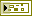error out contains error information. This output provides standard error out functionality.

## TSA Resampling (Equal)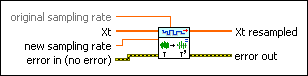original sampling rate specifies the original sampling rate of the equally-sampled time series Xt.Xt specifies the univariate time series.new sampling rate specifies the sampling rate for the resampled time series. The value must be greater than 0.error in describes error conditions that occur before this node runs. This input provides standard error in functionality.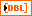Xt resampled returns the resampled univariate time series.error out contains error information. This output provides standard error out functionality.

## TSA Resampling (Unequal)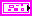Xt specifies the univariate time series.time index specifies the sampling time point of the unequally-sampled univariate time series Xt.data specifies the unequally-sampled data in the univariate time series Xt.new sampling rate specifies the sampling rate for the resampled time series. The value must be greater than 0.error in describes error conditions that occur before this node runs. This input provides standard error in functionality.Xt resampled returns the resampled univariate time series.error out contains error information. This output provides standard error out functionality.

## TSA Vector Resampling (Waveform)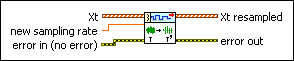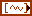Xt specifies the multivariate (vector) time series.new sampling rate specifies the sampling rate for the resampled time series. The value must be greater than 0.error in describes error conditions that occur before this node runs. This input provides standard error in functionality.Xt resampled returns the resampled multivariate (vector) time series.error out contains error information. This output provides standard error out functionality.

## TSA Vector Resampling (Equal)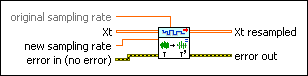original sampling rate specifies the original sampling rate of the equally-sampled time series Xt.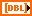Xt specifies the multivariate (vector) time series. Each column of the 2D array represents a vector at certain time.new sampling rate specifies the sampling rate for the resampled time series. The value must be greater than 0.error in describes error conditions that occur before this node runs. This input provides standard error in functionality.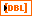Xt resampled returns the resampled multivariate (vector) time series. Each column of the 2D array represents a vector at certain time.error out contains error information. This output provides standard error out functionality.

## TSA Vector Resampling (Unequal)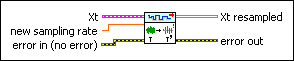Xt specifies the multivariate (vector) time series.time index specifies the sampling time point of the unequally-sampled multivariate (vector) time series Xt.data specifies the unequally-sampled data in the multivariate (vector) time series Xt. Each column of the 2D array represents a vector at certain time.new sampling rate specifies the sampling rate for the resampled time series. The value must be greater than 0.error in describes error conditions that occur before this node runs. This input provides standard error in functionality.Xt resampled returns the resampled multivariate (vector) time series. Each column of the 2D array represents a vector at certain time.error out contains error information. This output provides standard error out functionality.

## Examples

Refer to the following VIs for examples of using the TSA Resampling VI:

• Resample Time Series VI: labview\examples\Time Series Analysis\TSAGettingStarted
• Resample Unequally-Sampled Time Series VI: labview\examples\Time Series Analysis\TSAGettingStarted

Not Helpful